# Current Practice Level - 1

## 40 Questions MCQ Test Exclusive Video Lectures of Class 12 Physics by Experts | Current Practice Level - 1

Description
This mock test of Current Practice Level - 1 for Class 12 helps you for every Class 12 entrance exam. This contains 40 Multiple Choice Questions for Class 12 Current Practice Level - 1 (mcq) to study with solutions a complete question bank. The solved questions answers in this Current Practice Level - 1 quiz give you a good mix of easy questions and tough questions. Class 12 students definitely take this Current Practice Level - 1 exercise for a better result in the exam. You can find other Current Practice Level - 1 extra questions, long questions & short questions for Class 12 on EduRev as well by searching above.
QUESTION: 1

Solution:
QUESTION: 2

Solution:
QUESTION: 3

### A potential difference V is applied to a copper wire of length l and radius r. If length is doubled, the drift velocity

Solution:
QUESTION: 4

A potential difference V is applied to a copper wire of length l radius r. If radius is doubled, the drift velocity

Solution:
QUESTION: 5

When a motor car is started, its light becomes slightly dim because of

Solution:
QUESTION: 6

The belt of an elctrostatic generator is 50 cm wide and travels 30 cm/s. The belt carries charge into the sphere at a rate corresponding to 10-4 A. What is the surface density of charge on the belt.

Solution:
QUESTION: 7

Two wires of same dimensions but resistivities ρ1 an ρ2 are connected in series. The equivalent resistvity of the combination is

Solution:
QUESTION: 8

Equivalent resistance between A and B in the figure is: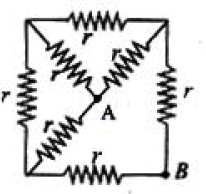Solution:
QUESTION: 9

Each of the resistors shown in the figure has resistance R. Find the equivalent resistance between A and B.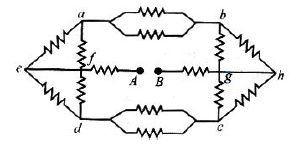Solution:
QUESTION: 10

Find the equivalent resistance between A and E (Resistance of each resistor is R.)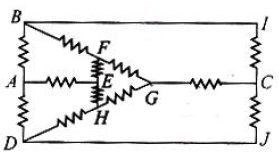Solution:
QUESTION: 11

The circuit shown has resistors of equal resistance R. Find the equivalent resistance between A and B, when the key is closed :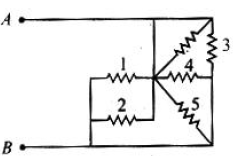Solution:
QUESTION: 12

In the circuit shown, current throught 25 V cell is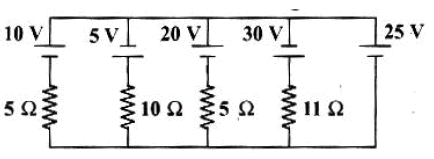Solution:
QUESTION: 13

A battery of internal resistance r having no load resistance has an e.m.f E volt. What is the observed e.m.f across the terminals of the battery when a load resistance R (= r) is connected to its terminals?

Solution:
QUESTION: 14

Figure represents a load consisting of three identical resistances connected to an electric energy source of e.m.f. 12 V and internal resistance 0.6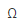. The ammeter reads 2A. The magnitude of each resistance is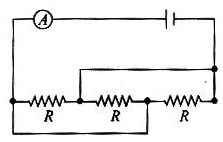Solution:
QUESTION: 15

Figure represents a part of closed circuit. The potential difference (VA- VB) is: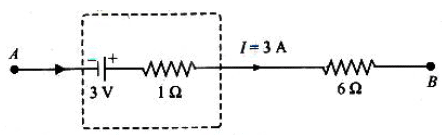Solution:
QUESTION: 16

Figure shows two squares, X and Y, cut from a sheet of metal, of uniform thickness t, X and Y have sides of length L and 2L, respectively.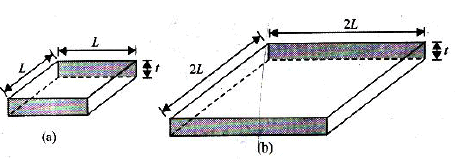The resistance Rx and Ry of the square are measured between the opposite faces shaded in Fig.What is the value of Rx / Ry ?

Solution:
QUESTION: 17

A cell on e.m.f E volt with no internal resistance is connected to a wire whose cross section changes. The wire has three sections of equal length. The middle section has a radius a, whereas the radius of the outer two sections is 2a. The ratio of the potential difference across section AB to the potential difference across section CA is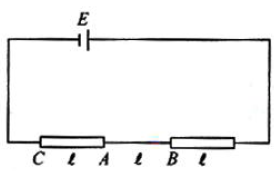Solution:
QUESTION: 18

Two cells A and B each of e.m.f 2 V, are connected in series to an external resistance R = 1. If the internal resistance of cells A is 1.9and that of B is 0.9, what is the potential difference between the terminals of cell A?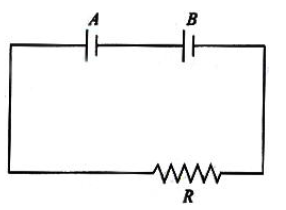Solution:
QUESTION: 19

Some early electric light bulbs used carbon filaments the resistance of which decreased as their temperature increased. Which of the following graphs best represents the way in which I, the current throught such a bulb, would depend upon V, the potential differed across it?

Solution:
QUESTION: 20

In the circuit shown in Fig. E1 = E2 = E3 = 2 V and R1 = R2 = 4. The current flowing between points A and B through battery E2 is: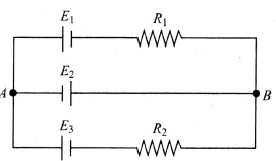Solution:
QUESTION: 21

An electric current flows along an insulated strip PQ of a metallic conductor. The current density in the strip varies as shown in the graph in Fig.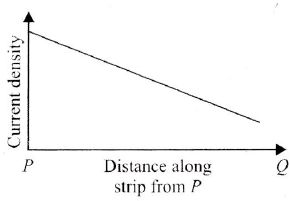Which of the following statements could explain this variation?

Solution:
QUESTION: 22

If a copper wire is stretched to make it 0.1% longer. The percentage change in its resistance is:

Solution:
QUESTION: 23

Resistance of a resistor at temperature t0C is Rt = R0 (1+ αt + βt2 ), where R0 is the resistance at 00C. The temperature coefficient of resistance at temperature t0C is

Solution:
QUESTION: 24

The mass of the three wires of copper are in the ratio 1 : 3 : 5. And their length are in?

Solution:
QUESTION: 25

A steady current flows in a metallic conductor of nonuniform cross section. The quantity/quantities constant along the length of the conductor is/are

Solution:
QUESTION: 26

In the part of circuit shown in Fig., the potential difference (VG - VH) between points G and H will be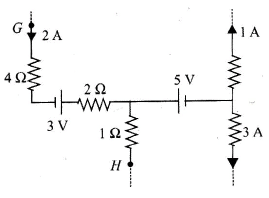Solution:
QUESTION: 27

The two ends of a uniform conductor with some resistance are joined to a cell of e.m.f E and some internal resistance r. Starting from the midpoint P of the conductor, we move in the direction of current and return to P while moving through the complete circuit. The potential V at every point on the path is plotted against the distance covered (x). Which of the following graphs best represents the resulting curve?

Solution:
QUESTION: 28

Find out the value of current throught 2resistance for the given circuit in Fig.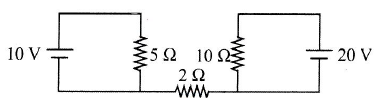Solution:
QUESTION: 29

A straight conductor of uniform cross section carries a current I. Let s be the specific charge of an electron. The momentum of all the free electrons per unit length of the conductor, due to their drift velocities only

Solution:
QUESTION: 30

The e.m.f of a cell is e and its internal resistance is r. Its terminal are connected to a resistance R. The potential difference between the terminals is 1.6 V for R = 4, and 1.8 V for R = 9. Then,

Solution:
QUESTION: 31

N identical cells are connected to form a battery. When the terminals of the battery are joined directly (shortcircuited), current I flows in the circuit. To obtain the maximum value of I,

Solution:
QUESTION: 32

n identical cells, each of e.m.f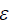and internal resistance r, are joined in series to form a closed circuit. One cell A is joined with re versed polarity. The potential difference across each cell, except A, is

Solution:
QUESTION: 33

n identical cells, each of e.m.fand internal resistance r, are joined in series to form a closed circuit. One cell A is joined with reversed polarity. The potential difference across A, is

Solution:
QUESTION: 34

Three resistance are joined together to form a letter Y, as shown in Fig. If the potentials of the terminals A, B and C are V1, V2 and Vrespectively, then determine the potential of the node O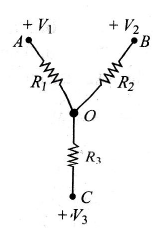Solution:
QUESTION: 35

In Fig., find the value of resistor to be connected between C and D, so that the resistance of the entire circuit between A and B does not change with the number of elementary sets.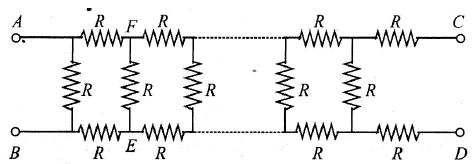Solution:
QUESTION: 36

For a cell, a graph is plotted between the potential difference V across the terminals of the cells and the current I drawn from the cell (see fig.) The e.m.f and the internal resistance of the cell are E and r, respectively. Then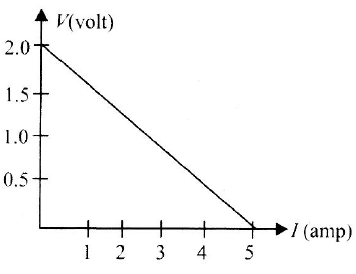Solution:
QUESTION: 37

A capacitor is charged and then made to discharge throught a resistance. The time constant is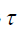. In what time will the potential difference across the capacitor decrease by 10%?

Solution:
QUESTION: 38

A capacitor is charged and then made to discharge throught a resistance. The time constant is. After how many time constants will the potential difference across the capacitor fall to 10% of its initial value?

Solution:
QUESTION: 39

A capacitor charges from a cell through a resistance. The time constant is. In what time will the capacitor collect 10% of its final charge?

Solution:
QUESTION: 40

A capacitor charges from a cell through a resistance. The time constant is. After how many time constants will the charge on the capacitor be 10% less than its final charge?

Solution: